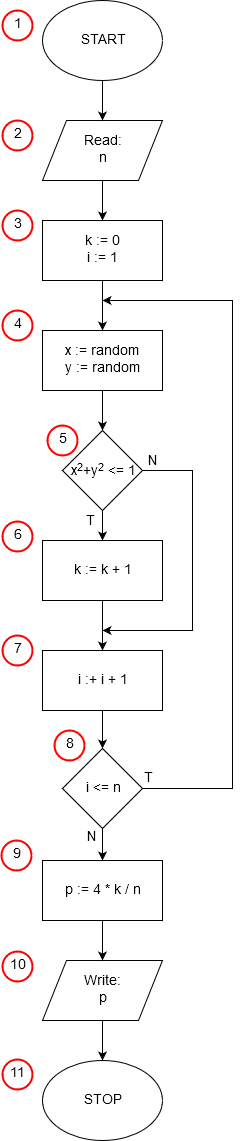# Monte Carlo Calculation of pi in Pythonimport random random.seed() n = int(input('n = ')) k = 0 for j in range(n): x = random.random() y = random.random() if x * x + y * y <= 1: k += 1 p = 4 * k / n print("pi =", p)